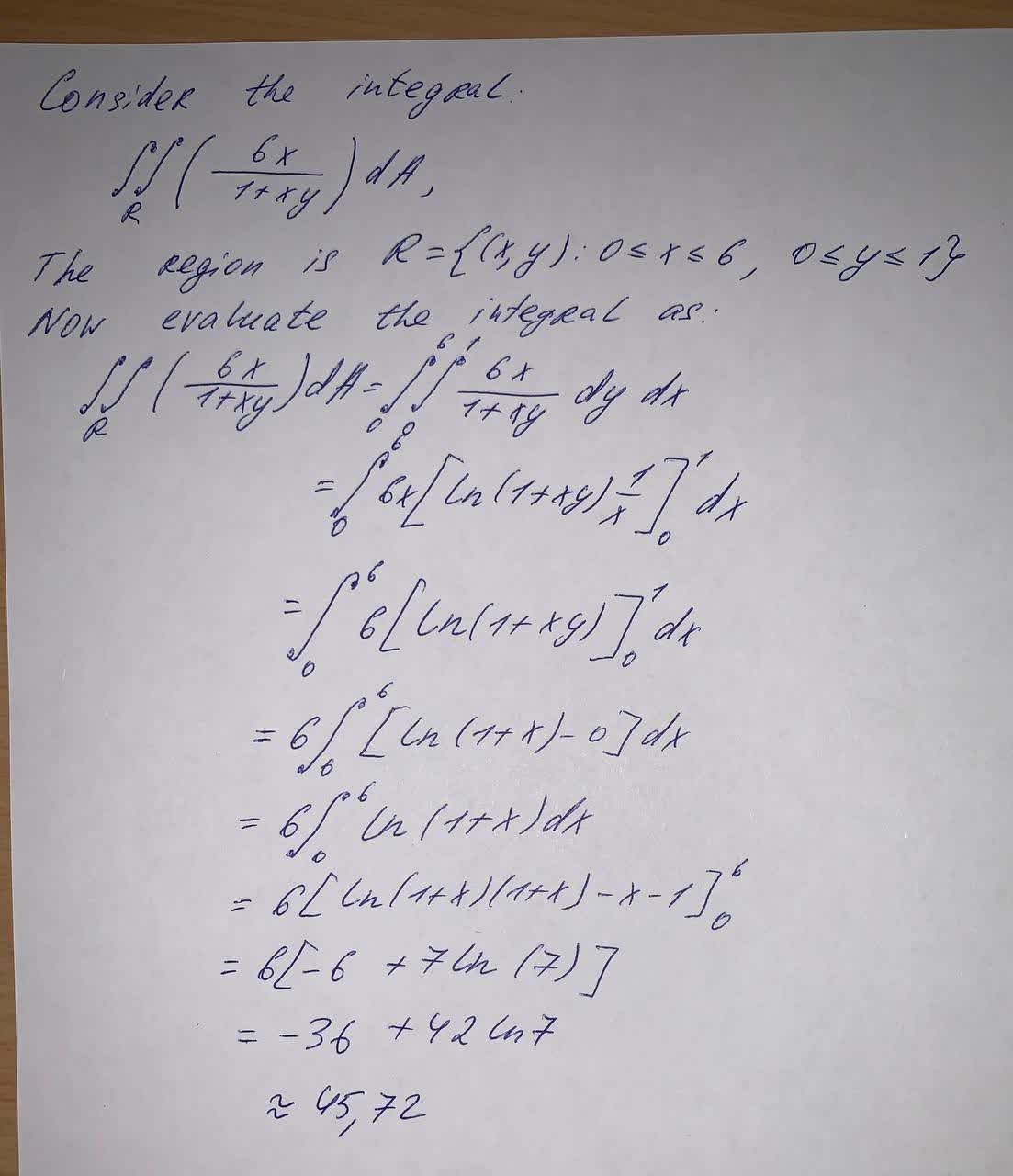# Calculate the double integral \frac{6x}{1+xy}dA , R=[0,6] \times [0,1]mattgondek4 2021-09-24 Answered
Calculate the double integral $$\displaystyle{\frac{{{6}{x}}}{{{1}+{x}{y}}}}{d}{A},{R}={\left[{0},{6}\right]}\times{\left[{0},{1}\right]}$$

• Questions are typically answered in as fast as 30 minutes

### Plainmath recommends

• Get a detailed answer even on the hardest topics.
• Ask an expert for a step-by-step guidance to learn to do it yourself.Mitchel Aguirre
Calculation: